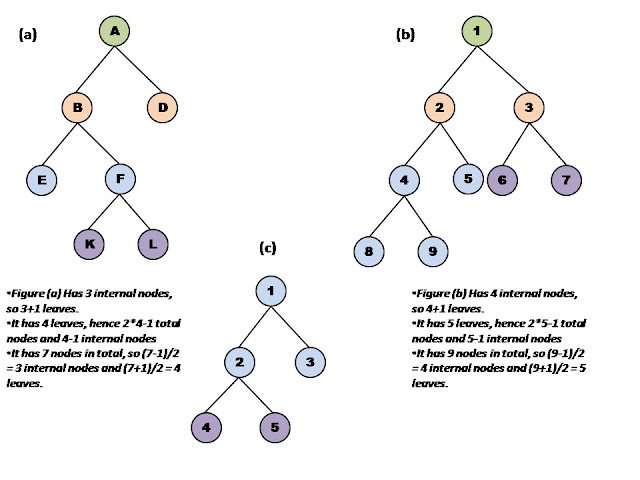Advanced Database Management System - Tutorials and Notes: Strict binary tree data structure

## Strict binary tree

A binary tree in which every node has either 0 or two children is called strict binary tree.

Properties:

• A strict binary tree with x internal nodes has exactly x+1 leaves.
• A strict binary tree with y external (leaf) nodes has (2y – 1) nodes (internal + external) and y-1 internal nodes exactly.
• A strict binary tree with n nodes (internal + external) has exactly (n-1)/2 internal nodes and (n+1)/2 external (leaf) nodes exactly.
You can work out these properties on the example trees given below;Strict Binary Trees
**********
Go to Binary Tree page

strict binary tree, define strict binary tree, example of strict binary tree, how does strict binary tree differ from other types of binary trees, differentiate between strict and full binary tree